.NET Framework 4.5.2+

# Row Interface

A row in a worksheet.

## Declaration

``````public interface Row :
CellRange,
Formatting,
IEnumerable<Cell>,
IEnumerable``````

## Remarks

A worksheet is divided into 1,048,576 rows and 16,384 columns. Use the Worksheet.Rows property to access the collection of worksheet rows.

### Access Rows

Use the RowCollection.Item property to access an individual row in a worksheet.

#### Obtain the Row at the Specified Index

``````using DevExpress.Spreadsheet;
// ...

// Access the row collection.
RowCollection rows = workbook.Worksheets.Rows;

// Access the first row in the collection.
Row firstRow = rows;
``````

A row index is zero-based. It specifies the row position in the collection.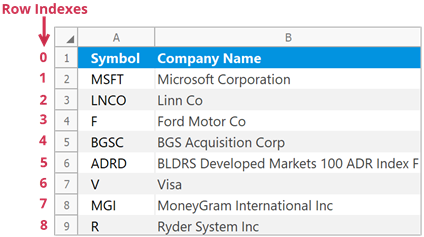#### Obtain the Row with a Given Heading

``````using DevExpress.Spreadsheet;
// ...

// Access the row collection.
RowCollection rows = workbook.Worksheets.Rows;

// Access the first row by its heading.
Row firstRow = rows["1"];
``````

Row headings help users identify each row in a worksheet.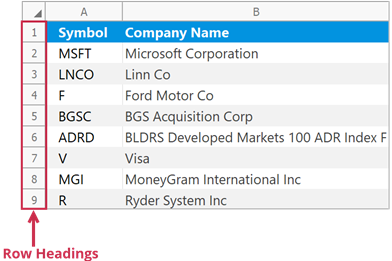### Insert Rows

Use the following methods to insert rows into a worksheet:

Row.Insert
Inserts a row above the current row.
RowCollection.Insert
Inserts rows at the specified position.

Note

The number of rows in a worksheet does not change - 1,048,576. When you add new rows, the rows below are shifted down and an equivalent number of rows at the end of the worksheet is removed.

#### Insert a Single Row

``````// Insert the third row.
worksheet.Rows["3"].Insert();

// Insert the fifth row.
worksheet.Rows.Insert(4);
``````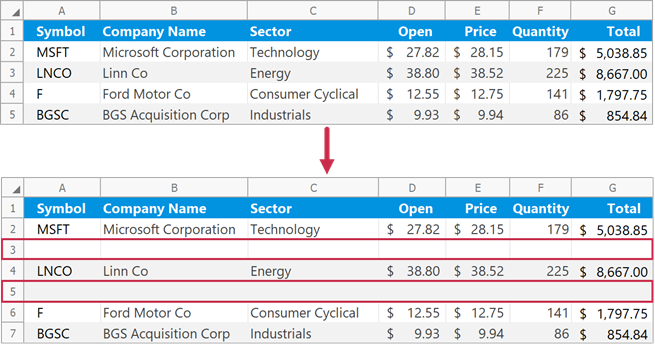#### Insert Multiple Rows

``````// Insert three rows (from row 3 to row 5).
worksheet.Rows.Insert(2, 3);
``````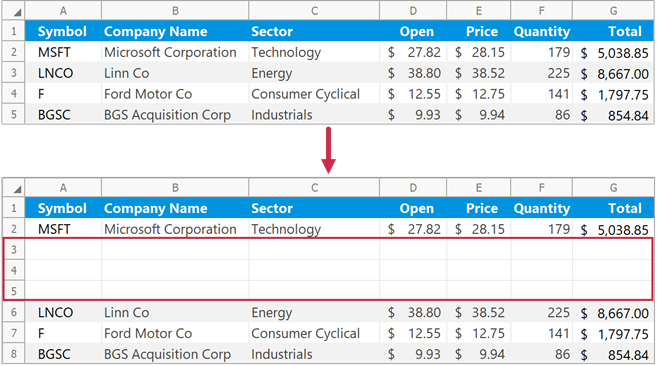#### Insert Rows Above a Specific Cell Range

Use the Worksheet.InsertCells method to insert empty rows above the specified cells. The method inserts the same number of rows as the specified cell range.

``````// Insert two rows above the "B3:E4" cell range.
worksheet.InsertCells(worksheet.Range["B3:E4"], InsertCellsMode.EntireRow);
``````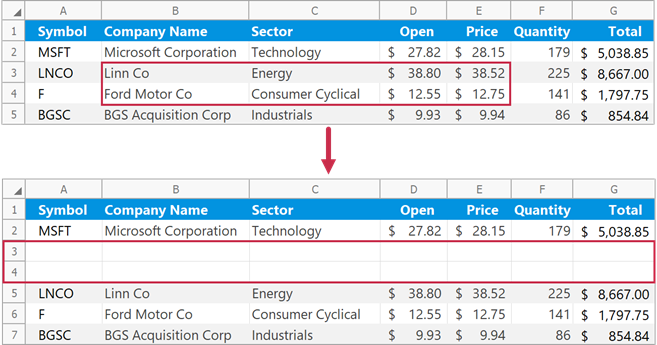#### Format Inserted Rows

Pass a RowFormatMode enumeration member to the RowCollection.Insert method to specify format options for inserted rows.

``````// Insert two rows with the same formatting as the first row.
worksheet.Rows.Insert(1, 2, RowFormatMode.FormatAsPrevious);
``````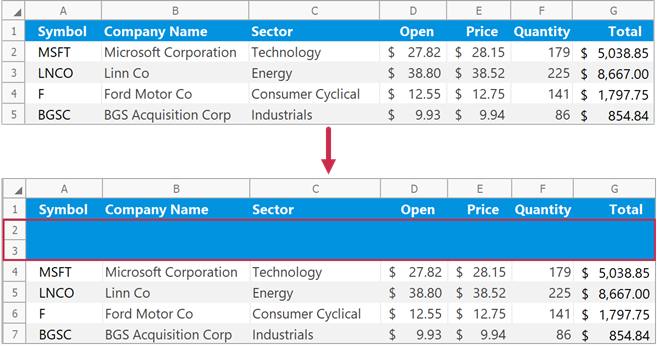### Remove Rows

Use the following methods to delete rows from a worksheet:

Row.Delete
Removes the current row.
RowCollection.Remove
Removes rows at the specified position.

Note

The number of rows in a worksheet does not change - 1,048,576. When you delete rows, the rows below are shifted up and an equivalent number of new rows is added to the end of the worksheet.

#### Remove a Single Row

``````// Delete the second row.
worksheet.Rows.Delete();

// Delete the third row.
worksheet.Rows.Remove(2);
``````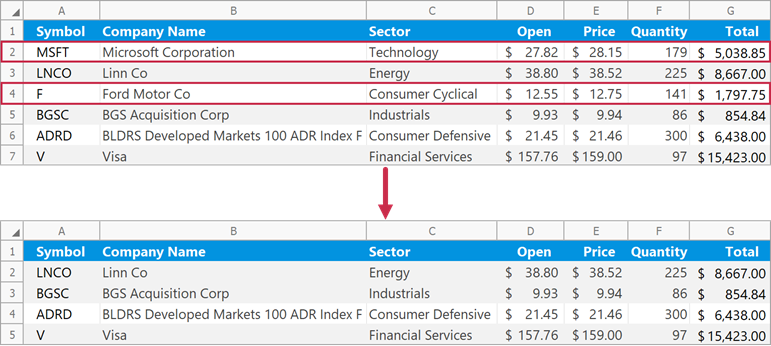#### Remove Multiple Rows

``````// Delete three rows (from row 2 to row 4).
worksheet.Rows.Remove(1, 3);
``````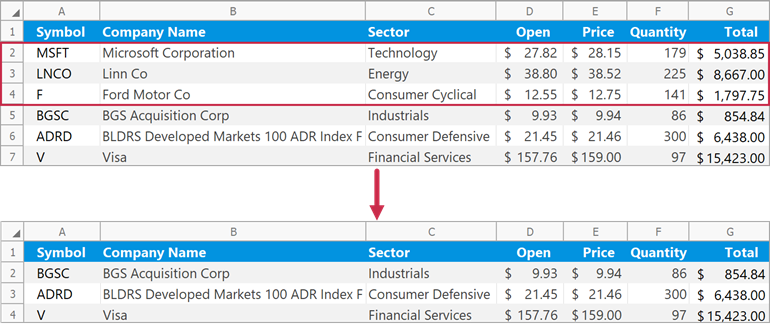#### Remove Rows That Meet a Specific Condition

``````// Specify the condition to remove worksheet rows.
// If a value in the "D" column is greater than 30, remove the corresponding row.
Func<int, bool> rowRemovalCondition = x => worksheet.Cells[x, 3].Value.NumericValue > 30.0;

// Delete rows that meet the specified condition.
// Check rows 2 through 7.
worksheet.Rows.Remove(1, 6, rowRemovalCondition);
``````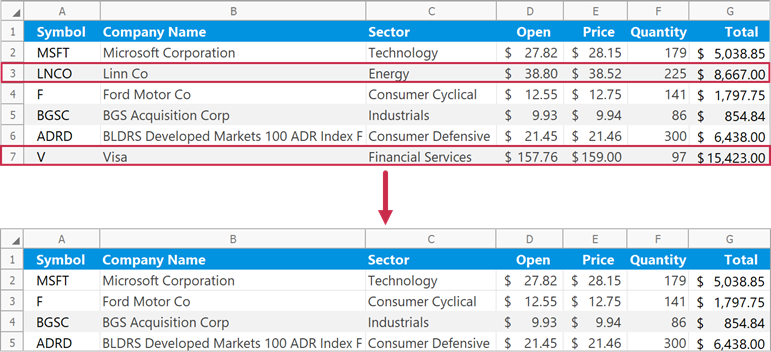#### Remove Rows That Contain Specific Cells

Use the Worksheet.DeleteCells method to delete rows that contain the specified cell range.

``````// Delete a row that contains the "B2" cell.
worksheet.DeleteCells(worksheet.Cells["B2"], DeleteMode.EntireRow);
``````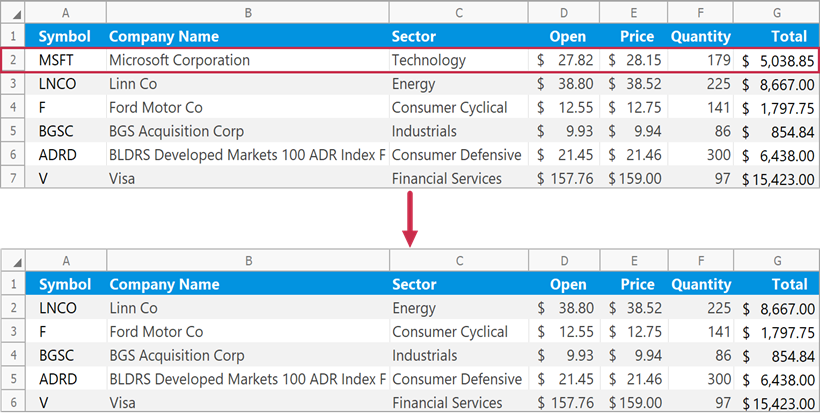### Group and Ungroup Rows

Use the RowCollection.Group method to group rows.

``````// Group rows 3 through 6 and collapse the group.
worksheet.Rows.Group(2, 5, true);

// Group rows 9 through 12 and expand the group.
worksheet.Rows.Group(8, 11, false);

// Group rows 2 through 13 to create the outer group.
worksheet.Rows.Group(1, 12, false);
``````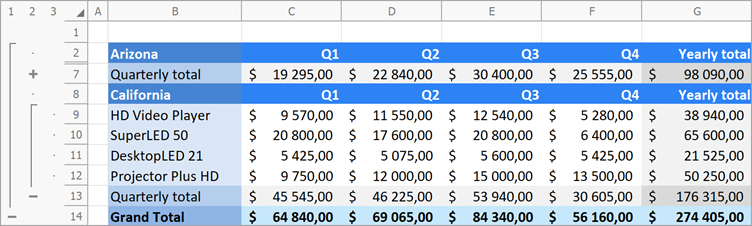Use the RowCollection.UnGroup method to ungroup rows.

``````// Ungroup rows 3 through 6 and display collapsed data.
worksheet.Rows.UnGroup(2, 5, true);

// Ungroup rows 9 through 12.
worksheet.Rows.UnGroup(8, 11, false);

// Remove the outer row group.
worksheet.Rows.UnGroup(1, 12, false);
``````

#### Expand or Collapse Groups

Use the following methods to collapse or expand row groups in a worksheet:

RowCollection.CollapseAllGroups
Collapses all row groups.
RowCollection.CollapseGroups
Collapses row groups starting with the specified group level.
RowCollection.ExpandAllGroups
Expands all row groups.
``````// Collapse all row groups except the first level group.
worksheet.Rows.CollapseGroups(2);
``````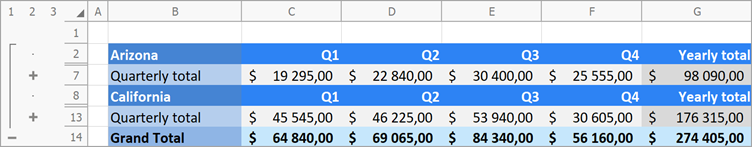### Hide Rows

Use the Row.Visible property or the RowCollection.Hide method to hide rows in a worksheet.

``````// Hide rows 2 through 6.
worksheet.Rows.Hide(1, 5);

// Hide row 8.
worksheet.Rows.Visible = false;
``````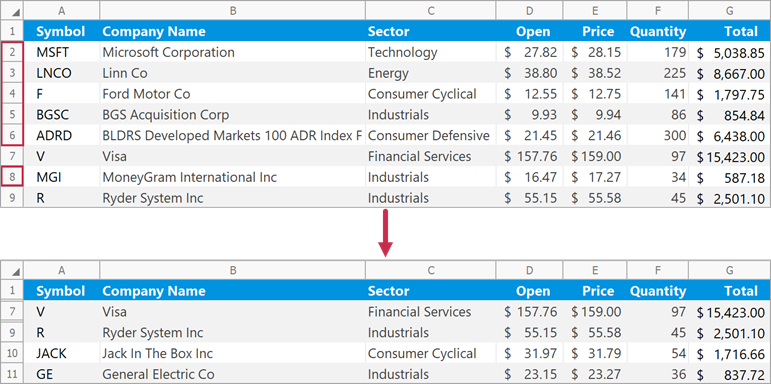Set the Row.Visible property to true to display a row. Use the RowCollection.Unhide method to show multiple hidden rows.

``````// Show rows 2 through 6.
worksheet.Rows.Unhide(1, 5);

// Show row 8.
worksheet.Rows.Visible = true;
``````

### Specify Row Height

#### AutoFit Row Height

Use the Row.AutoFit or RowCollection.AutoFit method to automatically change the row height to fit the contents.

``````// Adjust the height of row 2.
worksheet.Rows.AutoFit();

// Adjust the height of rows 3 through 5.
worksheet.Rows.AutoFit(2, 4);
``````

#### Set the Height Value

Use the Row.Height property to specify the row height in measurement units defined by the Workbook.Unit property.

``````// Set the height of row 2 to 50 points.
workbook.Unit = DevExpress.Office.DocumentUnit.Point;
worksheet.Rows.Height = 50;

// Set the height of row 5 to the height of row 2.
worksheet.Rows["5"].Height = worksheet.Rows["2"].Height;
``````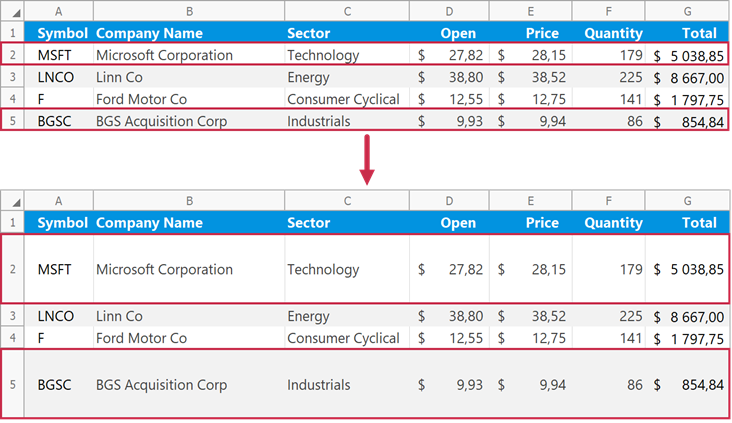Note

If the row height is set to 0, the row is hidden. You can also use the Row.Visible property to hide a row or display the hidden row again.

#### Set the Default Row Height

Use the Worksheet.DefaultRowHeight property to set the default height for worksheet rows.

Note

The Worksheet.DefaultRowHeight property does not affect rows with explicitly specified heights.

``````// Set the default row height to 30 points.
workbook.Unit = DevExpress.Office.DocumentUnit.Point;
worksheet.DefaultRowHeight = 30;
``````

#### Set Row Height for a Cell Range

Use the CellRange.RowHeight property to set the height of rows that contain the specified cell range.

``````// Specify the height of the row that contains the "C5" cell.
workbook.Unit = DevExpress.Office.DocumentUnit.Inch;
worksheet.Cells["C5"].RowHeight = 0.5;
``````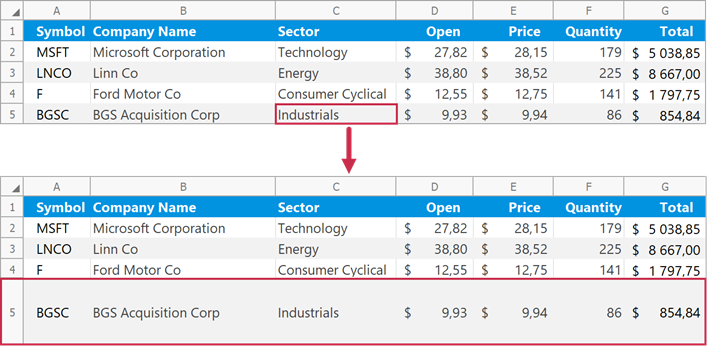#### Set the Height of All Rows

Use the Column.RowHeight property to specify the height of all rows in a worksheet.

``````// Set the height of all rows to 40 points.
workbook.Unit = DevExpress.Office.DocumentUnit.Point;
worksheet.Columns.RowHeight = 40;
``````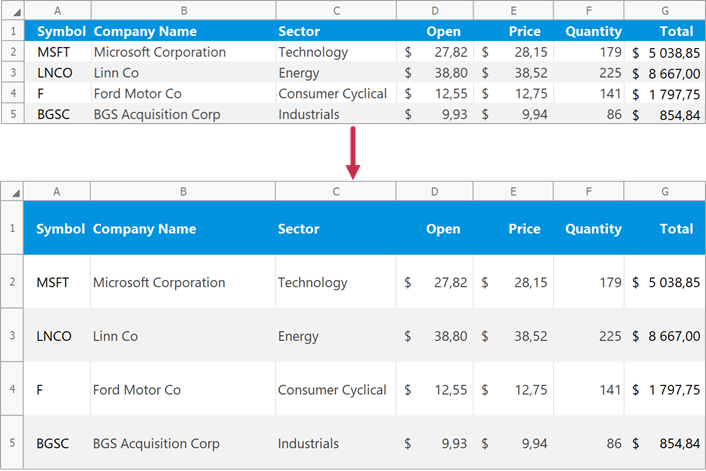### Copy Rows

Use the Row.CopyFrom method to copy a row in a worksheet.

``````// Copy data from row 3 to row 2.
worksheet.Rows.CopyFrom(worksheet.Rows);
``````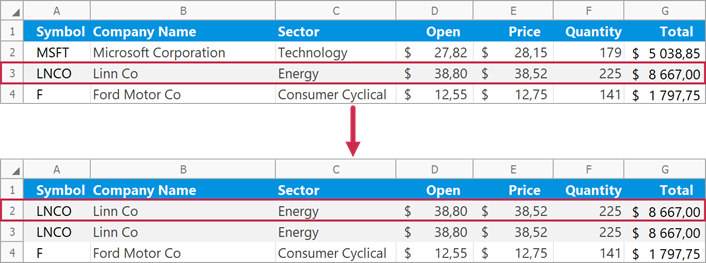Pass a PasteSpecial enumeration member to the Row.CopyFrom method to specify which part of the copied data should be pasted into destination cells.

``````// Copy number formats from row 3 to row 2.
worksheet.Rows.CopyFrom(worksheet.Rows, PasteSpecial.NumberFormats);
``````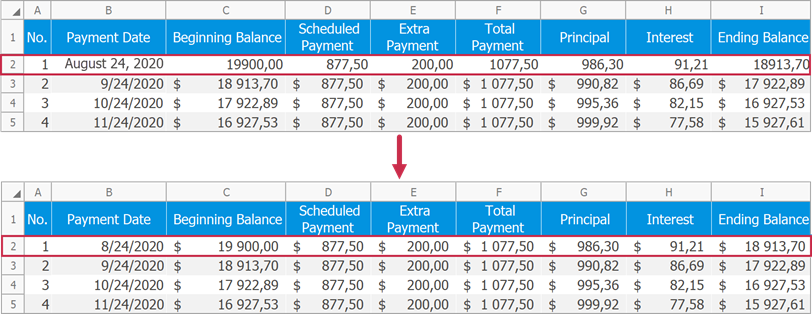### Move Rows

Use the Row.MoveTo method to move a row to a different position in a worksheet.

Note

When you move rows, existing destination cell content is replaced.

``````// Move row 3 up one row.
worksheet.Rows.MoveTo(worksheet.Rows);
``````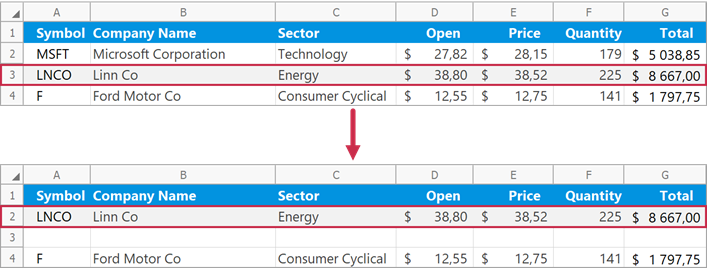Show 35 items